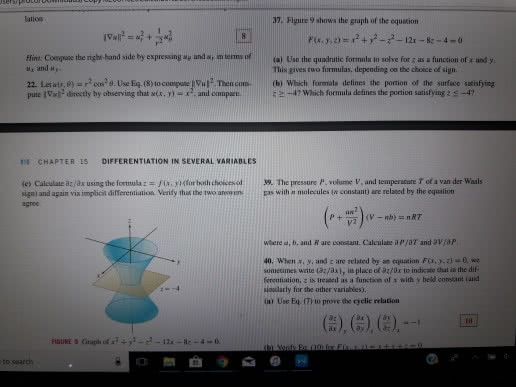1
0
watching
87
views13 Nov 2019

##37 please tation 37. Figure 9 shows the graph of the equation F(x. y, z) =x2 + y2ã¼ãº2-121-k-4-0 Hint: Coespuse the right-band side by expressing ag and wr im terns ofa) Use the quadratic formula to sohve for z as a function of x and y M and uy 22. Letit, d)=r2 cos28. Use Eq (8)tocon pite â½"12 Then con- (b) which formula defines the portion of the surface satisfying pute Vl directly by observing that a(x, y) - x7, and compare. This gives Ewo formulas, depending on the choice of sign 4? Which formula defines the portion satisfying z s-47 E CHAPTER 15 DIFFERENTIATION IN SEVERAL VARIABLES (c, Calculate az ax using the formula z = f(x y)(for both doi esat 39. The pressure P. volume V, and temperature T of a van der Waals sigm) and again via implicil differentiation. Verify thas the two aneswreras with a molecules iw constant) are relabed by the equation P + v2 where a, b, and R are constant. Calculate d P/aT and aVjo P 40. When x, y, and z are related by an equation Fix. y.2)- 0, we sometimes write (32/3x), in place of azfax to indicate that in the dif ferentiation, z is treased as a function of s with y held constant (and siooilarly for the other variables). (a) Use Eq (T) to prove the gelic relation ax to search